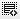## How are intangibles assets valued ?0
How are intangibles assets valued ??0

Usually a company's intangible assets are valued by subtracting a firm's book value from its market value.
Due to market value constantly changes, the value of intangible assets changes also, making it an inferior measure.
Finding a company's CIV involves seven steps:
1. Calculate the average pretax earnings for the past three years.
2. Calculate the average year-end tangible assets for the past three years.
3. Calculate the company's return on assets (ROA).
4. Calculate the industry average ROA for the same three-year period as in Step
5. Calculate excess ROA by multiplying the industry average ROA by the average tangible assets calculated in Step 2. Subtract the excess return from the pretax earnings from Step 1.
6. Calculate the three-year average corporate tax rate and multiply by the excess return. Deduct the result from the excess return.
7. Calculate the net present value of the after-tax excess return. Use the company's cost of capital as a discount rate.

Click on thisicon to add code snippet.